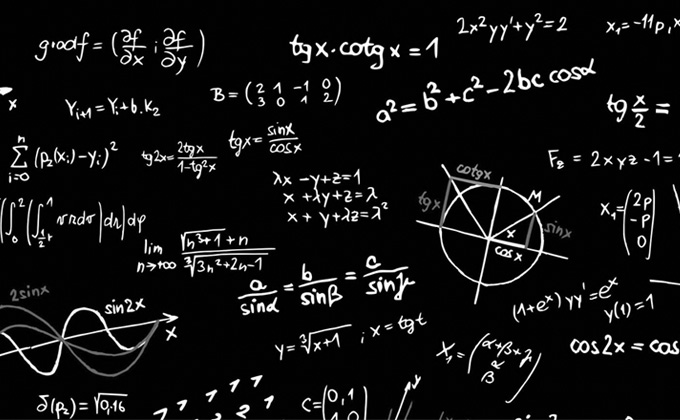# Quant section will be trickyCAT Notification: August 2023This year too in CAT 2021, QA (Quantitative Ability)will be a separate section which will comprise of 34 questions with a stipulated time of 60 minutes. The exam is scheduled to be conducted in November 2021

If there is something that can upset the strong will and confidence of even the most serious CAT aspirants it would definitely be the Quantitative Ability (QA).

There is no any fix syllabus for this section but with the expert analysis of previous CAT papers, following topics are of utmost importance:

Number System

• Number Base System
• BODMAS
• HCF/LCM
• AP/GP
• AM/GM/HM
• Central Tendency (Mean/Median/Mode)
• Average
• Ratio and Proportion
• Percentage

Algebra

• Equation
• In-Equation

Time -Speed ,Distance and Time, Profit and Loss, Geometry etc.

Practice Question

Question

In the figure given below, AD = AE = 4 cm and BD = CE = 2 cm. If BC is a straight line and ∠ADE = 60°, find the length of AB.SolutionGet Complete Information on CAT 2021 Application Form